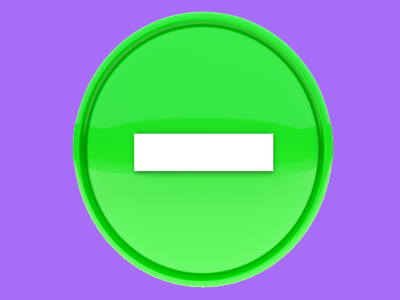Difference, subtract, subtraction and minus are all words which mean take away.

The Language of Subtraction

This Math quiz is called 'The Language of Subtraction' and it has been written by teachers to help you if you are studying the subject at elementary school. Playing educational quizzes is a fun way to learn if you are in the 1st or 2nd grade - aged 6 to 8.

It costs only \$12.50 per month to play this quiz and over 3,500 others that help you with your school work. You can subscribe on the page at Join Us

In elementary school, children extend their understanding of the language of subtraction to include 'difference'. They should already know and be using words such as subtract, subtraction, take away and minus. Recognizing the language of subtraction is essential in order to be able to make decisions about which operation to use to solve calculations and problems.

Can you recognize the subtraction words?

1.
What is 16 take away 6?
16
6
26
10
'Take away' is another way of saying subtract
2.
How much is 6 less than 18?
12
8
9
15
'Less than' is a subtraction word - you could also count backwards
3.
Which word could be used in this number sentence?
12 ___ 5 = 7
subtract
plus
total
Subtract means to take away
4.
Which of the following is not a subtraction word?
Take away
Subtract
Multiply
Minus
Multiply does not mean subtract
5.
What is the difference between 12 and 5?
One is odd, one is even
7
One is 2-digit, the other isn't
17
The 1st and 3rd options are differences but in math 'difference' means subtract. To find the difference, subtract the smaller number from the larger one
6.
Which word could be used in this number sentence?
15 ___ 9 = 6
plus
divided by
minus
Minus means to subtract
7.
I have 15 cakes and eat 6. How many are left?
6
9
8
4
'How many left' is a clue that this is a subtraction
8.
17 minus which number = 5?
7
12
5
9
Minus means to take away. 17 - 12 = 5
9.
What is the symbol for subtraction?
-
x
+
=
This sign means to subtract or take away
10.
8 is the difference between which 2 numbers?
2 and 4
12 and 20
6 and 10
12 and 14
To find the difference, subtract the smaller number from the larger one
Author:  Angela Smith

We use cookies to make your experience of our website better.

To comply with the new e-Privacy directive, we need to ask for your consent -